# Physics Final Part b short Answer

 Physics Final Part B Short Answer 1. What is a motion diagram? 2. What is the significance of defining a coordinate system to study the motion of an object? 3. How is displacement different from distance? Under what conditions can an object travel a certain distance and yet its resultant displacement be zero? 4. What is the equation of motion for average velocity? 5. Define average velocity. 6. The position-time graph of an object is found to be a straight line passing through the origin. What information about the motion of the object is provided by the graph? 7. Given below is the position-time graph representing motions of two runners, Nick and Ian. Use this graph to determine which runner has greater average velocity.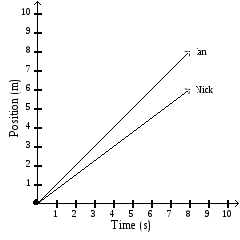8. Given below is the particle model of a boy skating on a smooth, pedestrian-free sidewalk. The time interval between successive dots is 2 s.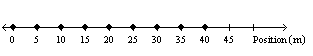Plot a position-time graph to represent the motion of the boy. 9. The position-time graph given below represents the motion of Ted returning home from the market on his bike. What is the similarity between his displacement and the average velocity?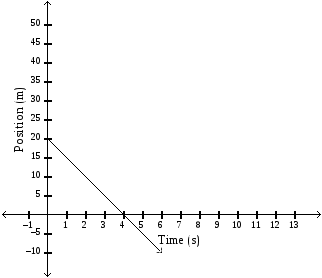10. Given below is the motion diagram of a small ball rolling straight on a frictionless surface.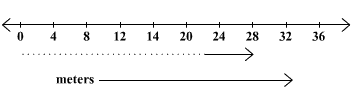The time interval between successive positions is 1 s. a. What is the displacement of the ball after 3 s? b. Where does the ball reach after 5 s? c. After rolling for 7 s, the direction of motion of the ball is changed. It starts rolling toward its starting point. Assume the coordinate system and the speed of the ball to remain unchanged. What is the displacement of the ball between 7 s and 9 s? 11. Given below is the graph representing the position-time graphs of two swimmers (A and B), swimming in a pool along a straight line. Both the swimmers start from two different positions. Use the graph to find when and where swimmer B passes swimmer A.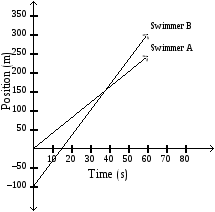12. The position-time graph of a pedestrian is given below. What is his displacement after 2.5 s?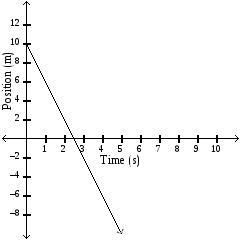13. What information is provided by the points on the line of a position-time graph of an object? Download 0.53 Mb.Share with your friends: